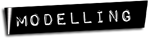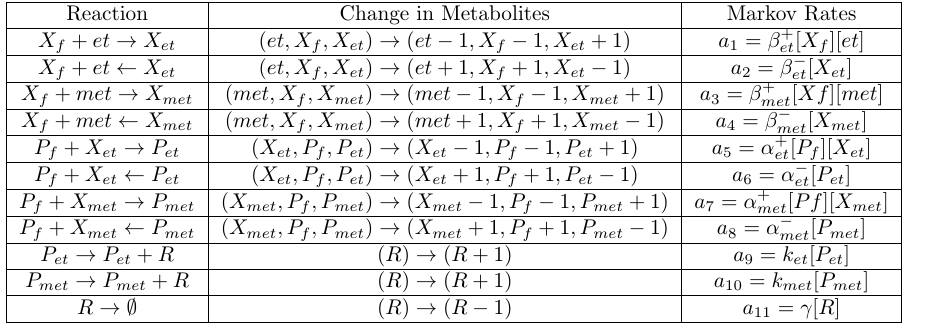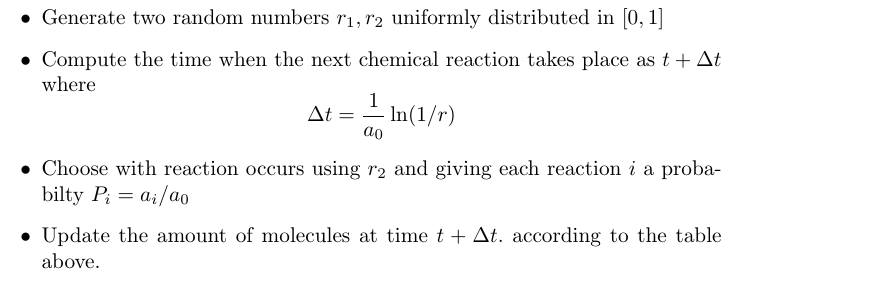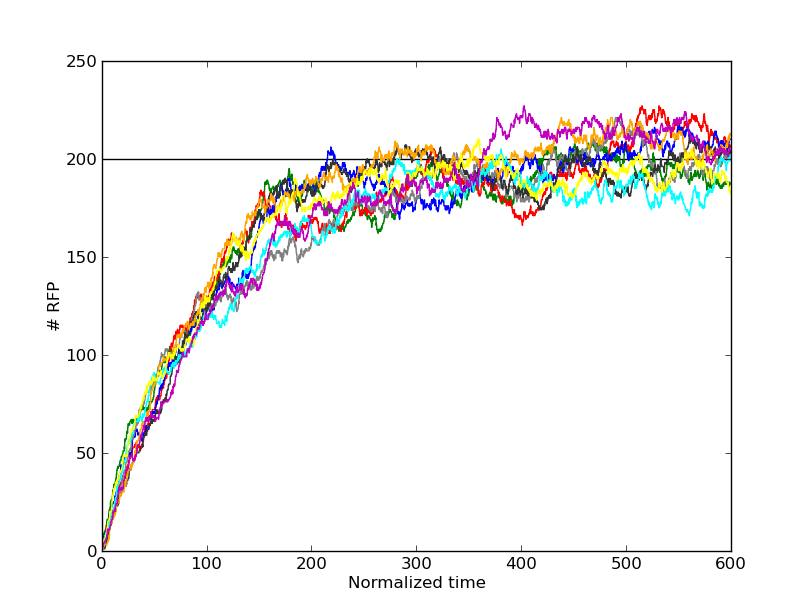# Team:USP-Brazil/Model:Stochastic## Stochastic model

### Introduction

We want to simulate a cell where is happening all the chemical reactions discribed in the Deterministic Model as a Stochastical Process whose states are determinated by a collection of nine numbers:

\begin{equation} (et, met, X_f,X_{et}, X_{met}, P_f,P_{et}, P_{met}, R) \end{equation}

Those numbers represent the amount of mollecules of each chemical species in the cell. Respectively, the amount of ethanol, methanol, free transcription factor, ethanol-binded transcription factor, methanol-binded transcription factor, free promoter, ethanol-binded promoter, methanol-binded promoter and RFP molecules inside the cell.

### Continuous Time Markov Chain

The main idea behind the Stochastic Modeling is that given an chemical reaction, e.g.: $$A \longrightarrow B$$ A single mollecule will react after some time t, following a probability distribution of the form $$p(t) = \lambda e^{-\lambda t}$$

These are the only kind of distribution in continuous time which do not have a "memory" or in Mathematical language:

\begin{equation} P(X (t+\delta t) = i | X(t) = j) =P(X (\delta t) = i | X(0) = j) \end{equation}

So the probability does not depend of which states the system was in the time interval [0,t], that is, the future state of the system only depends on its present state. A larger explanation of that is in Chapter 5 of .

When there are [A] os molecules "trying" to react the first reaction will occur with a probability distribution (as we see on sections 5.4.1 and 5.7.3 in ) given by:

$$p(t) = [A] \lambda e^{- [A] \lambda t}$$

We can see that this λ parameter really looks like a parameter of a deterministic equation: $$\frac{d[A]}{dt} = - k_A [A]$$

Analising that the mean life time must be the same in both, to fit the same kind of experimental results, we realized that:

\begin{equation} \lambda = k_A \end{equation}

### Our Circuit by Markov

\begin{equation} X_f + et \rightleftharpoons X_et \end{equation}

The Probability of each reaction happens before a time t follows the distribution:

\begin{equation} P((et,Xf,Xet) \rightarrow (et-1,Xf-1,Xet+1),t)= { \beta_{et}^{+}[Xf][et]} e^{- \beta_{et}^{+}[Xf][et] t} \end{equation} $$P((et,Xf,Xet) \rightarrow (et+1,Xf+1,Xet-1) ,t)= { \beta_{et}^{-}[Xet]}e^{- \beta_{et}^{-}[Xf][et] t}$$

As we explained before, the Rates βet. is the same one from the Deterministic model.

All reactions:If we have some reactions happening at the rate

$$p_i(t) = - a_i e^{-a_i t}$$

Then the probability of a reaction occurring at a time previous to tA is:

\begin{equation} P_i(t \leq t_A)= \int_0^{t_A} p_i(t) = 1 - e^{-a_i t} \end{equation}

And the probability that the reaction does not occour before this time is:

$$P_i(t \geq t_A) = 1 - P(t \leq t_A)= e^{-a_i t}$$

Being all the reactions independents, the probability of no reaction occurring before a given time point tA is equal to the probability all reactions occurring before tA . That is:

$$P_0(t \geq t_A) = \prod_{i=1}^{11} P_i(t \geq t_A) = \prod_{i=1}^{11} e^{- a_i t_A}$$

Defining

F$$a_0 = \sum_{i=1}^{11} a_i$$

So we realize that the probability of one reaction occurring at a time before tA is

$$P_0(t \geq t_A) = e^{a_0 t_A}$$

And therefore the time until the first reaction will be given by the distribution:

$$p(t) = - a_0 e^{a_0t}$$r, uniformly distributed in [0,1]. The quantity

$$\Delta t = \frac{1}{a_0}\ln (1/r)$$ has a probability distribution given by: $$p(\Delta t) = - a_0 e^{a_0t}$$

Once the the time t + Δ t when the next reaction occurs is calculated, all that remains is to choose which reaction should happen. Another random variable uniformly distributed in [0,1] is used, and the probability correspondent to each reaction i is

$$P_i = a_i/a_0$$.

### Guilespe

This stochastic simulation was implemented by the Gillespie algorithm, described above and well explained in . This algorithm consists in repeating the instructions below beginning at some initial condition for the metabolites, and updating the amount of each kind of mollecule after each time interval.### Simulated

The code for the implementation of our algorithm is available at: https://dl.dropboxusercontent.com/u/23798199/gillespie.py.

The results are shown in the graph below.Results of stochastic simulation.

Different colours represent different cells been simulated at the same initial condition. In this example, the initial conditions are given by:

$$(et, met, X_f,X_{et}, X_{met}, P_f,P_{et}, P_{met}, R) (t=0) = (10000,10000,100,0,0,2,0,0,0)$$

It is important to note that, unlike in the deterministic model, no hypothesis were made. This model does not suppose that: (i)The equilibrium for the transcript factor product is reached much faster than any protein production. (ii)The intracellular concentration of ethanol and methanol does not change trouth the process due to Xf being much lower than [et] and [met]. (iii) The promoter is bound most of the time.

Even without these hypotesis, equlibrium was reached at a value of RFP concentration very close to the indicated by the deterministic model, which is indicated by a black line in the graph. In this way, the predictions and the assumptions of the deterministic model are corroborated by a more comprehensive stochastic model.

\begin{equation} \end{equation} \begin{equation} \end{equation}

#### References

 Sheldon M. Ross, Stochastic Process, Wiley, New York 1996

 Radek Erban, S. Jonathan Chapman, Philip K. Maini: A prac tical guide to stochastic simulations of reaction-diffusion processes , http://arxiv.org/abs/0704.1908

RFP Visibility | Deterministic Model | Stochastic Model